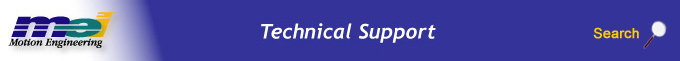.

# PT and PVT Path Motion

## Introduction

This document describes the position-time (PT) and position-velocity-time (PVT) path interpolation algorithms for the XMP controller. A technical description of each algorithm pointing out the advantages and disadvantages is contained in the following sections of this document.

## General Characteristics

PT and PVT algorithms convert a series of point-time pairs into XMP motion frames that create the real-time command positions at each sample during the time intervals between the point-time pairs. The PVT interpolation type requires additional data at each point (the vector velocity). A “point” in these point-time series may have several dimensions (axes).

## PT Algorithm

The PT algorithm fits a simple constant velocity profile between the user specified “Position and Time” points. The PT algorithm guarantees that the XMP’s trajectory calculator will exactly hit each specified position at the specified time. The constant velocity segment is simply calculated by the difference of the positions divided by the time interval: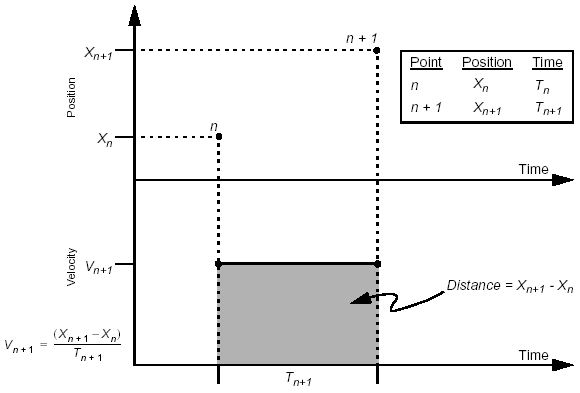PT algorithm

### Simple PT Example

For example, a trapezoidal velocity profile motion could be generated using a list of Position and Time points: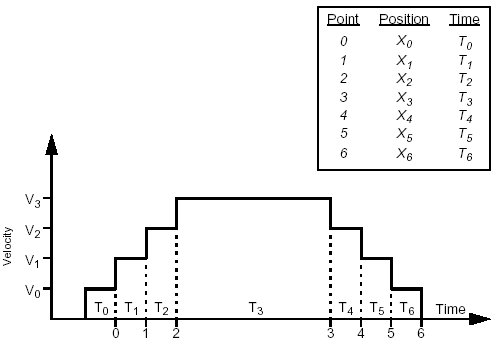PT profile (trapezoidal)

### When is the PT algorithm useful?

The PT algorithm is very good for closely spaced points or low velocities. It is a very simple algorithm, requiring very few calculations; therefore, it is fast. It works well with low performance motion systems. If the points are spaced too far apart, the motion will be rough, because the acceleration between each point is instantaneous. It is best to keep the point spacing within a few samples. The XMP-Series default sample rate is 500 microseconds.

## PVT Algorithm

The PVT algorithm fits a Jerk (non-constant acceleration) profile between user specified "Position, Velocity, and Time" points. The PVT algorithm guarantees that the XMP's trajectory calculator will exactly hit each specified position, with each specified velocity, at the specified time.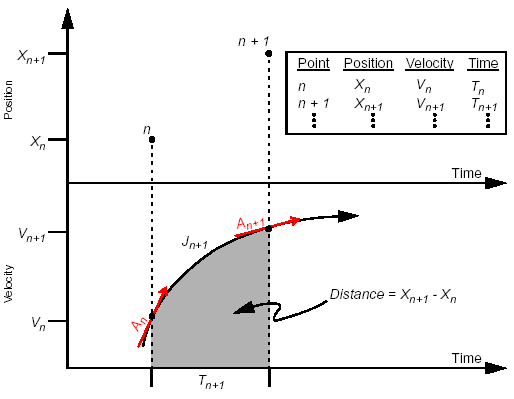PVT algorithm

For each point, the PVT algorithm calculates the Acceleration and Jerk values to exactly hit the specified Position and Velocity at the next point. The equations for An and Jn, are derived from the standard kinematic equations: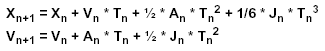The derivation of the kinematic equations in terms of An and Jn is beyond the scope of this document.

## Simple PVT Example

For example, a trapezoidal velocity profile motion could be generated using a list of three Position, Velocity, and Time points: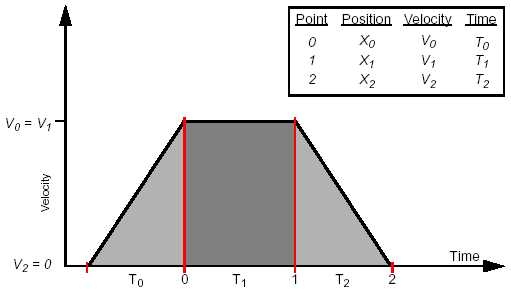PVT profile (trapezoidal)

How do you calculate the Velocities for PVT paths? There are several methods to calculate the velocities, depending on the desired path profile. Here are some common methods:

 Calculate the change in distance between two points divided by the time: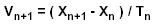This is a simple method, with relatively smooth performance. You will need to add an extra final point to the list, setting the last velocity to zero. Calculate a constant acceleration fit between points. From the kinematic equations (with Jerk = 0), we know: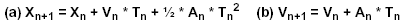substitute (b) into (a), and solve for Vn+1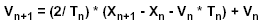There are several other methods that can be used to calculate the velocities. Additionally, there are many other algorithms, like averaging and splines that can be applied to PVT.

### What happens if the Velocities are calculated improperly for PVT paths?

The PVT algorithm doesn’t care if the Position, Velocity, Time points are matched properly. The PVT algorithm will simply calculate a Jerk profile between points to reach the specified point. The profile between the points may not be desired, but it will be accurate.

For example, consider the simple three point trapezoidal profile from the PVT profile (trapezoidal) graph above. If the velocities at points 0 and 1 were too small, the profile would stretch to cover the proper distance (area under the curve):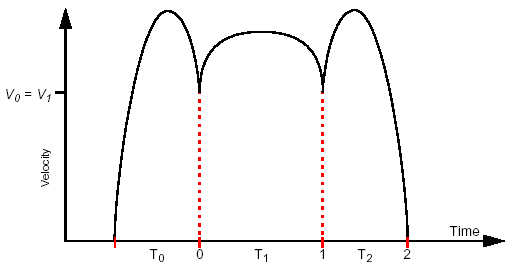PVT profile, velocities too low

If the velocities at points 0 and 1 were too big, the profile would shrink to cover the proper distance (area under the curve):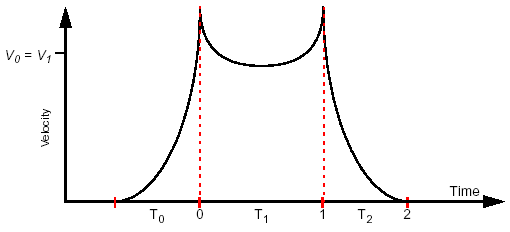PVT profile, velocities too high

### When is the PVT algorithm useful?

The PVT algorithm is very good for smooth and close path control. The points can be spaced very close or very far apart. For complex paths (or path portions) the points should be spaced close together. For simple paths (or path portions) the points can be spaced far apart. PVT can handle virtually any list of points. The most difficult part is determining the appropriate velocities at each point.

## Test Cases

Three types of point-time series (step, zigzag, and octagon) were used to evaluate the paths created by the PT/PVT algorithms. Most higher order (polynomial) interpolation algorithms are better suited for describing continuously curved sections of paths than sections with straight lines and sharp corners. The step and zigzag path tests determine how well an algorithm behaves when the path was not very appropriate for high-order polynomials. The octagon path tests how well the algorithms interpolate circular sections of a path given a small number (8) of points around the circle.

The simplest path studied was a one-dimensional step. The following point-time series was used to test the interpolation:

Point-time Series for Testing Interpolation
 Time (samples) Position (counts) Velocity (PVT only) 0 0 0 500 0 0 1000 0 0 1500 0 0 2000 5000 0 2500 5000 0 3000 5000 0 3500 5000 0

The step is useful for determining if the algorithm is going to have problems in sections of a path with sharp corners. The amount of overshoot (if any), the closeness to lines joining the points, and accelerations and velocities near the step can be evaluated. The step is also useful for determining how much of the path will be affected by a sharp corner (at what distance from the step does the path return to a straight line). For example steps affect Cubic spline interpolation over the entire path, where PT interpolation is only affected at the two points closest to the step.

The zigzag path (really a reversed “Z”) was used to evaluate sharp corner behavior in a two-dimensional (X-Y) path. The following point list formed the zigzag path:

 Time (samples) X,Y (counts) Vx, Vy (counts/sec.,PVT only) 0 0,0 0,0 500 5000,0 20000,0 1000 0,10000 20000,0 1500 5000,1000 0,0

The octagon path determines how well the interpolation algorithms are suited for circular sections of a path. The nine points along the path are on a circle with a radius of 5000 counts, centered at 0,5000. The first and last points are the same (at 0,0 the 6 o’clock position) and the points are spaced at angles of 45 degrees.

## PT Interpolation

This is the simplest form of interpolation. The interpolation is linear (first order). While the path is mathematically continuous, velocity, acceleration, and jerk are not. The lines between points are straight and are traversed at constant speed. PT motion will usually be rough unless the points are very closely spaced in time or the velocities are very low.

### Step behavior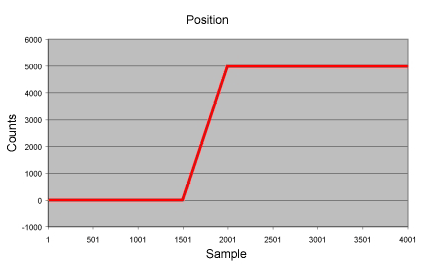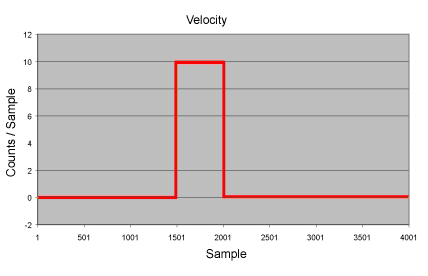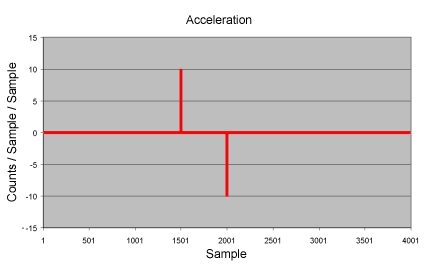PT motion is well suited for paths composed of straight lines and sharp corners. The only serious problem with PT motion is the large number of points required to limit the peak accelerations. In this example the peak acceleration was 40 million c/sec/ sec.

### Zigzag path behavior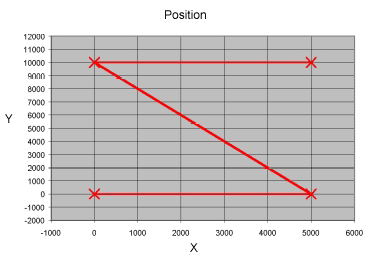The path is simply straight lines joining the points.

### Octagon path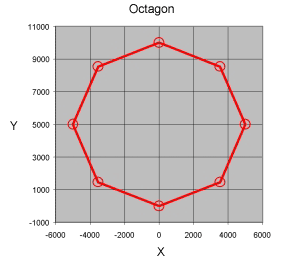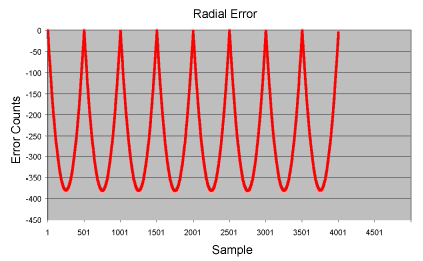Again, the path consists of simple straight lines. Radial error is the difference between he distance from the interpolated path to the center from the radius of a circle through the points. The largest error is midway between the points.

## PVT Interpolation

PVT paths are third order (cubic) rather than first order (ex: PT paths). The position and velocity are continuous, the acceleration and jerk are not. PVT paths tend to be much smoother than PT paths, but the velocity of each axis needs to be provided at each of the supplied points. This can increase the complexity of the application since the velocity at each point is often difficult to determine.

### Step behavior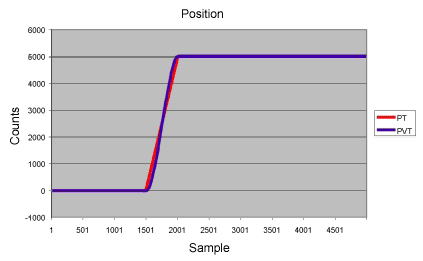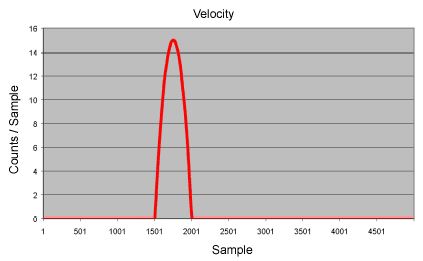### Zigzag behavior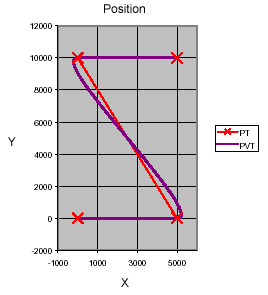### Octagon behavior: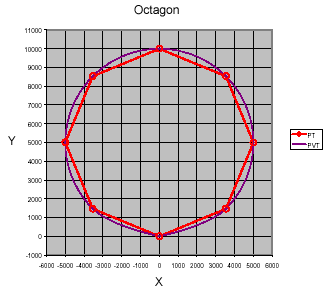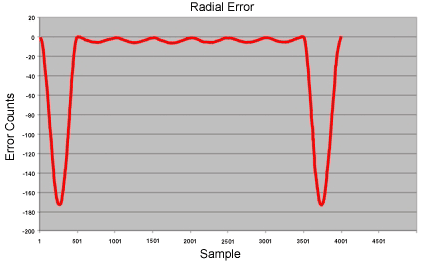The larger error in the first and last segments is caused by the V=0 constraints at
the end points.

 Legal Notice  |  Tech Email  |  Feedback        Copyright © 2001-2021 Motion Engineering